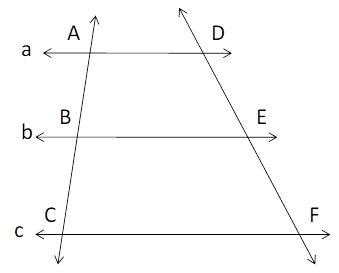Chapter 4.4, Problem 26E### Elementary Geometry for College St...

6th Edition
Daniel C. Alexander + 1 other
ISBN: 9781285195698

#### Solutions

Chapter
Section### Elementary Geometry for College St...

6th Edition
Daniel C. Alexander + 1 other
ISBN: 9781285195698
Textbook Problem
1 views

# In the figure, a | | b | | c and B is the midpoint of A C ¯ . If A B = 2 x + 3 y , B C = x + y + 7 and D E = 2 x + 3 y + 3 and E F = 5 x − y + 2 , Find x         a n d           y .Exercises 25, 26

To determine

To Find:

The value of xandy.

Explanation

The given diagram is

Given: a||b||c and B is the midpoint of AC¯.

Also, AB=2x+3y, BC=x+y+7 and DE=2x+3y+3 and EF=5xy+2.

We know that, when three or more parallel lines intercept congruent line segments on one transversal, then they intercept congruent line segments on any transversal.

Therefore, ABBCandDEEF.

Now, 2x+3y=x+y+7.

It becomes 2x+3yxy=7.

Then x+2y=7.

Therefore, x=72y

### Still sussing out bartleby?

Check out a sample textbook solution.

See a sample solution

#### The Solution to Your Study Problems

Bartleby provides explanations to thousands of textbook problems written by our experts, many with advanced degrees!

Get Started

#### Find more solutions based on key concepts